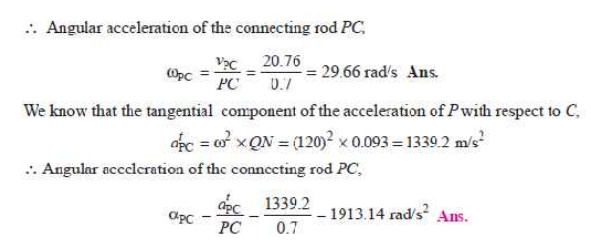Home | | Dynamics of Machines | Klien‚Äôs Construction

# Klien‚Äôs ConstructionKlien‚Äôs construction: 1 Klien‚Äôs velocity diagram, 2 Klien‚Äôs acceleration diagram

KLIEN‚ÄôS CONSTRUCTION

Let OC be the crank and PC the connecting rod of a reciprocating steam engine, as shown in Fig. 15.2 (a). Let the crank makes an angle Œ∏ with the line of stroke PO and rotates with uniform angular velocity œâ rad/s in a clockwise direction. The Klien‚Äôs velocity and acceleration diagrams are drawn as discussed below:Fig. 15.2. Klien‚Äôs construction.

1 Klien‚Äôs velocity diagram

First of all, draw OM perpendicular to OP; such that it intersects the line PC produced at M. The triangle OCM is known asKlien‚Äôs velocity diagram. In this triangle OCM,

OM may be regarded as a line perpendicular to PO,

CM may be regarded as a line parallel to PC, and ...(Q It is the same line.)

CO may be regarded as a line parallel to CO.

We have already discussed that the velocity diagram for given configuration is a triangle ocp as shown in Fig. 15.2 (b). If this triangle is revolved through 90¬∞, it will be a triangle oc1 p1, in which oc1 represents vCO (i.e. velocity of C with respect to O or velocity of crank pin C) and is paralel to OC,

op1 represents vPO (i.e. velocity of P with respect to O or velocity of cross-head or piston P) and is perpendicular to OP, and c1p1 represents vPC (i.e. velocity of P with respect to C) and is parallel to CP.

A little consideration will show, that the triangles oc1p1 and OCM are similar. Therefore,Thus, we see that by drawing the Klien‚Äôs velocity diagram, the velocities of various points may be obtained without drawing a separate velocity diagram.

2 Klien‚Äôs acceleration diagram

The Klien‚Äôs acceleration dia-gram is drawn as discussed below:

1.                   First of all, draw a circle with C as centre and CM as radius.

2.                   Draw another circle with PC as diameter. Let this circle inter-

sect the previous circle at K  and L.

3. Join KL and produce it to intersect PO at N. Let KL intersect PC at Q.

This forms the quadrilateral CQNO, which is known as Klien‚Äôs acceleration diagram.

We have already discussed that the acceleration diagram for the given configuration is as shown in Fig. 15. 2 (c). We know thatA little consideration will show that the quadrilateral o'c'x p' [Fig. 15.2 (c)] is similar to quadrilateral CQNO [Fig. 15.2 (a)]. Therefore,SOLVED PROBLEMS

1.                        The crank and connecting rod of a reciprocating engine are 200 mm and 700 mm respectively. The crank is rotating in clockwise direction at 120 rad/s. Find with the help of Klein‚Äôs construction: 1. Velocity and acceleration of the piston, 2. Velocity and acceleration of the mid point of the connecting rod, and 3. Angular velocity and angular acceleration of the connecting rod, at the instant when the crank is at 30¬∞ to I.D.C. (inner dead centre).

Solution. Given: OC = 200 mm = 0.2 m ; PC = 700 mm = 0.7 m ; œâ = 120 rad/sThe Klein‚Äôs velocity diagram OCM and Klein‚Äôs acceleration diagram CQNO as shown in Fig. 15.5 is drawn to some suitable scale, in the similar way as discussed in Art. 15.5. By measurement, we find that

OM = 127 mm = 0.127 m ; CM = 173 mm = 0.173 m ; QN = 93 mm = 0.093 m ; NO = 200 mm = 0.2 m

1. Velocity and acceleration of the piston

We know that the velocity of the piston P,

vP = œâ √ó OM = 120 √ó 0.127 = 15.24 m/s Ans. and acceleration of the piston P,

aP = œâ 2 √ó NO = (120)2 √ó 0.2 = 2880 m/s 2  Ans.

2. Velocity and acceleration of the mid-point of the connecting rod

In order to find the velocity of the mid-point D of the connecting rod, divide CM at D1 in the same ratio as D divides CP. Since D is the mid-point of CP, therefore D1 is the mid-point of CM, i.e. CD1 = D1M. Join OD1. By measurement,

OD1 = 140 mm = 0.14 m

Velocity of D, vD = œâ √ó OD1 = 120 √ó 0.14 = 16.8 m/s Ans.

In order to find the acceleration of the mid-point of the connecting rod, draw a line DD2 parallel to the line of stroke PO which intersects CN at D2. By measurement,

OD2 = 193 mm = 0.193 m ‚à¥ Acceleration of D,

aD = œâ 2 √ó OD2 = (120)2 √ó 0.193 = 2779.2 m/s 2 Ans. 3. Angular velocity and angular acceleration of the connecting rod

We know that the velocity of the connecting rod PC (i.e. velocity of P with respect to C), vPC = œâ √ó CM = 120 √ó 0.173 = 20.76 m/sStudy Material, Lecturing Notes, Assignment, Reference, Wiki description explanation, brief detail
Mechanical : Dynamics of Machines : Force Analysis : Klien‚Äôs Construction |